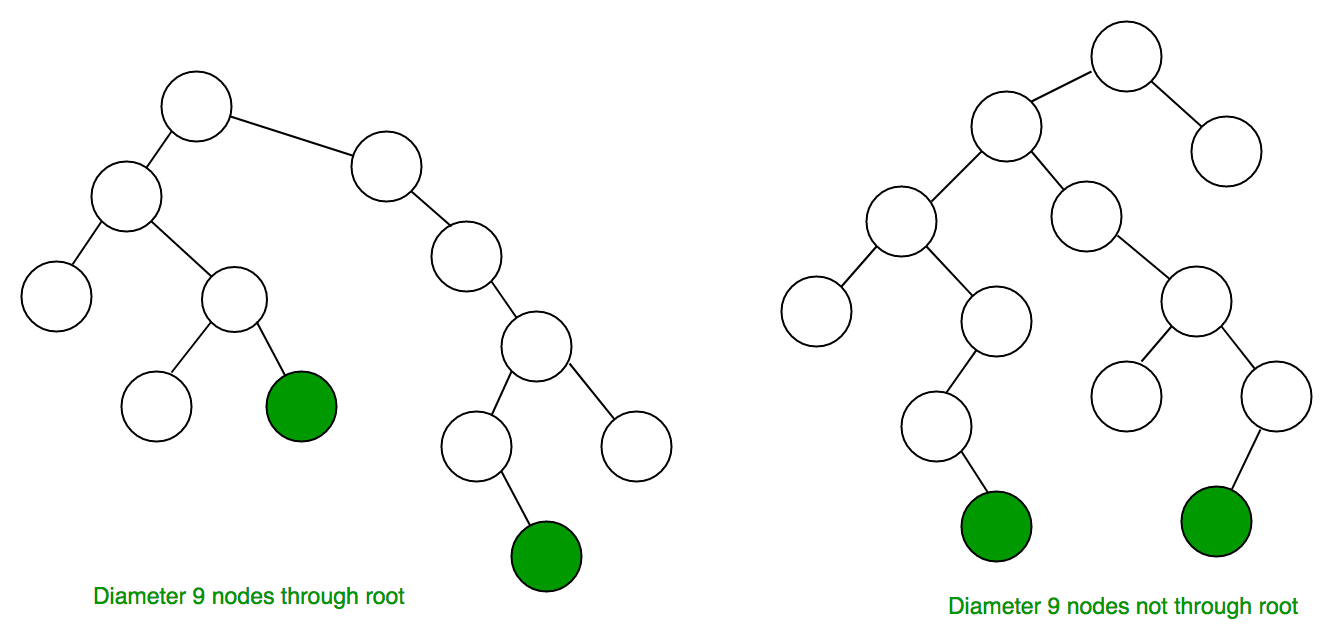GeeksforGeeks App
Open AppBrowser
Continue

# Diameter of a Binary Tree in O(n) [A new method]

The diameter of a tree is the number of nodes on the longest path between two leaves in the tree. The diagram below shows two trees each with diameter nine, the leaves that form the ends of the longest path are colored (note that there may be more than one path in the tree of the same diameter).Examples:

```Input :     1
/   \
2      3
/  \
4     5

Output : 4

Input :     1
/   \
2      3
/  \ .    \
4     5 .    6

Output : 5```

We have discussed a solution in below post.
Diameter of a Binary Tree

In this post a new simple O(n) method is discussed. Diameter of a tree can be calculated by only using the height function, because the diameter of a tree is nothing but maximum value of (left_height + right_height + 1) for each node. So we need to calculate this value (left_height + right_height + 1) for each node and update the result. Time complexity – O(n)

Implementation:

## C++

 `// Simple C++ program to find diameter``// of a binary tree.``#include ``using` `namespace` `std;` `/* Tree node structure used in the program */``struct` `Node {``    ``int` `data;``    ``Node* left, *right;``};` `/* Function to find height of a tree */``int` `height(Node* root, ``int``& ans)``{``    ``if` `(root == NULL)``        ``return` `0;` `    ``int` `left_height = height(root->left, ans);` `    ``int` `right_height = height(root->right, ans);` `    ``// update the answer, because diameter of a``    ``// tree is nothing but maximum value of``    ``// (left_height + right_height + 1) for each node``    ``ans = max(ans, 1 + left_height + right_height);` `    ``return` `1 + max(left_height, right_height);``}` `/* Computes the diameter of binary tree with given root. */``int` `diameter(Node* root)``{``    ``if` `(root == NULL)``        ``return` `0;``    ``int` `ans = INT_MIN; ``// This will store the final answer``    ``height(root, ans);``    ``return` `ans;``}` `struct` `Node* newNode(``int` `data)``{``    ``struct` `Node* node = ``new` `Node;``    ``node->data = data;``    ``node->left = node->right = NULL;` `    ``return` `(node);``}` `// Driver code``int` `main()``{``    ``struct` `Node* root = newNode(1);``    ``root->left = newNode(2);``    ``root->right = newNode(3);``    ``root->left->left = newNode(4);``    ``root->left->right = newNode(5);` `    ``printf``(``"Diameter is %d\n"``, diameter(root));` `    ``return` `0;``}`

## Java

 `// Simple Java program to find diameter``// of a binary tree.``class` `GfG {` `/* Tree node structure used in the program */``static` `class` `Node``{``    ``int` `data;``    ``Node left, right;``}` `static` `class` `A``{``    ``int` `ans = Integer.MIN_VALUE;``}` `/* Function to find height of a tree */``static` `int` `height(Node root, A a)``{``    ``if` `(root == ``null``)``        ``return` `0``;` `    ``int` `left_height = height(root.left, a);` `    ``int` `right_height = height(root.right, a);` `    ``// update the answer, because diameter of a``    ``// tree is nothing but maximum value of``    ``// (left_height + right_height + 1) for each node``    ``a.ans = Math.max(a.ans, ``1` `+ left_height +``                    ``right_height);` `    ``return` `1` `+ Math.max(left_height, right_height);``}` `/* Computes the diameter of binary``tree with given root. */``static` `int` `diameter(Node root)``{``    ``if` `(root == ``null``)``        ``return` `0``;` `    ``// This will store the final answer``    ``A a = ``new` `A();``    ``int` `height_of_tree = height(root, a);``    ``return` `a.ans;``}` `static` `Node newNode(``int` `data)``{``    ``Node node = ``new` `Node();``    ``node.data = data;``    ``node.left = ``null``;``    ``node.right = ``null``;` `    ``return` `(node);``}` `// Driver code``public` `static` `void` `main(String[] args)``{``    ``Node root = newNode(``1``);``    ``root.left = newNode(``2``);``    ``root.right = newNode(``3``);``    ``root.left.left = newNode(``4``);``    ``root.left.right = newNode(``5``);` `    ``System.out.println(``"Diameter is "` `+ diameter(root));``}``}` `// This code is contributed by Prerna Saini.`

## Python3

 `# Simple Python3 program to find diameter``# of a binary tree.` `class` `newNode:``    ``def` `__init__(``self``, data):``        ``self``.data ``=` `data``        ``self``.left ``=` `self``.right ``=` `None` `# Function to find height of a tree``def` `height(root, ans):``    ``if` `(root ``=``=` `None``):``        ``return` `0` `    ``left_height ``=` `height(root.left, ans)` `    ``right_height ``=` `height(root.right, ans)` `    ``# update the answer, because diameter``    ``# of a tree is nothing but maximum``    ``# value of (left_height + right_height + 1)``    ``# for each node``    ``ans[``0``] ``=` `max``(ans[``0``], ``1` `+` `left_height ``+``                             ``right_height)` `    ``return` `1` `+` `max``(left_height,``                   ``right_height)` `# Computes the diameter of binary``# tree with given root.``def` `diameter(root):``    ``if` `(root ``=``=` `None``):``        ``return` `0``    ``ans ``=` `[``-``999999999999``] ``# This will store``                          ``# the final answer``    ``height_of_tree ``=` `height(root, ans)``    ``return` `ans[``0``]` `# Driver code``if` `__name__ ``=``=` `'__main__'``:``    ``root ``=` `newNode(``1``)``    ``root.left ``=` `newNode(``2``)``    ``root.right ``=` `newNode(``3``)``    ``root.left.left ``=` `newNode(``4``)``    ``root.left.right ``=` `newNode(``5``)` `    ``print``(``"Diameter is"``, diameter(root))` `# This code is contributed by PranchalK`

## C#

 `// Simple C# program to find diameter``// of a binary tree.``using` `System;` `class` `GfG``{` `/* Tree node structure used in the program */``class` `Node``{``    ``public` `int` `data;``    ``public` `Node left, right;``}` `class` `A``{``    ``public` `int` `ans = ``int``.MinValue;``}` `/* Function to find height of a tree */``static` `int` `height(Node root, A a)``{``    ``if` `(root == ``null``)``        ``return` `0;` `    ``int` `left_height = height(root.left, a);` `    ``int` `right_height = height(root.right, a);` `    ``// update the answer, because diameter of a``    ``// tree is nothing but maximum value of``    ``// (left_height + right_height + 1) for each node``    ``a.ans = Math.Max(a.ans, 1 + left_height +``                    ``right_height);` `    ``return` `1 + Math.Max(left_height, right_height);``}` `/* Computes the diameter of binary``tree with given root. */``static` `int` `diameter(Node root)``{``    ``if` `(root == ``null``)``        ``return` `0;` `    ``// This will store the final answer``    ``A a = ``new` `A();``    ``int` `height_of_tree = height(root, a);``    ``return` `a.ans;``}` `static` `Node newNode(``int` `data)``{``    ``Node node = ``new` `Node();``    ``node.data = data;``    ``node.left = ``null``;``    ``node.right = ``null``;` `    ``return` `(node);``}` `// Driver code``public` `static` `void` `Main()``{``    ``Node root = newNode(1);``    ``root.left = newNode(2);``    ``root.right = newNode(3);``    ``root.left.left = newNode(4);``    ``root.left.right = newNode(5);` `    ``Console.WriteLine(``"Diameter is "` `+ diameter(root));``}``}` `/* This code is contributed by Rajput-Ji*/`

## Javascript

 ``

Output

`Diameter is 4`

Time complexity: O(n) ,  where n is number of nodes in binary tree .
Auxiliary Space: O(h) for call stack , where h is height of binary tree .

This article is contributed by Pooja Kamal. If you like GeeksforGeeks and would like to contribute, you can also write an article using write.geeksforgeeks.org or mail your article to review-team@geeksforgeeks.org. See your article appearing on the GeeksforGeeks main page and help other Geeks.

My Personal Notes arrow_drop_up